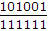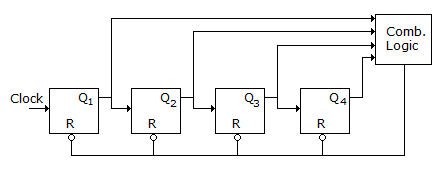# Electronics and Communication Engineering - Digital Electronics

41.

Out of 5 M x 8, 1 M x 16, 2 M x 16 and 3M x 8 memories, which memory can store more bits?

 A. 5 M x 8 B. 2 M x 16 C. 3 M x 8 D. 1 M x 16

Answer: Option A

Explanation:

Bits are 40 M, 16 M and 32 M.

42.

A 6 bit ladder A/D converter has input 101001. The output is (assume 0 = 0 V and 1 = 10 V)

 A. 4.23 B. 6.41 C. 5.52 D. 9.23

Answer: Option B

Explanation:

Output =10 j 6.41 V.

43.

A ripple counter has 4 bits and uses flip flops with propagation delay time of 25 ns. The maximum possible time for change of state will be

 A. 25 ns B. 50 ns C. 75 ns D. 100 ns

Answer: Option D

Explanation:

In ripple counter all the delays are added.

44.

A counter has a modulus of 10. The number of flip flops is

 A. 10 B. 5 C. 4 D. 3

Answer: Option C

Explanation:

23 = 8 and 24 = 16 Therefore 4 flip-flops are needed. Some states will be skipped to give a modulus of 10.

45.

The counter shown in the given figure is built using 4 -ve edge triggered toggle FFs. The FF can be set asynchronously when R = 0. The combinational logic required to realize a modulo-13 counter isA. F = Q4 Q3 Q2 Q1 B. F = Q4 + Q3 + Q2 + Q1 C. F = Q4 + Q3 + Q2 + Q1 D. F = Q4Q3Q2Q1

Answer: Option A

Explanation:

Counter is modulo-13, it will count up to 15 but due to mod-13, it will be reset at 13, (13)10 = (1101 )2 = Q4 Q3 Q2 Q1.

#### Current Affairs 2021

Interview Questions and Answers